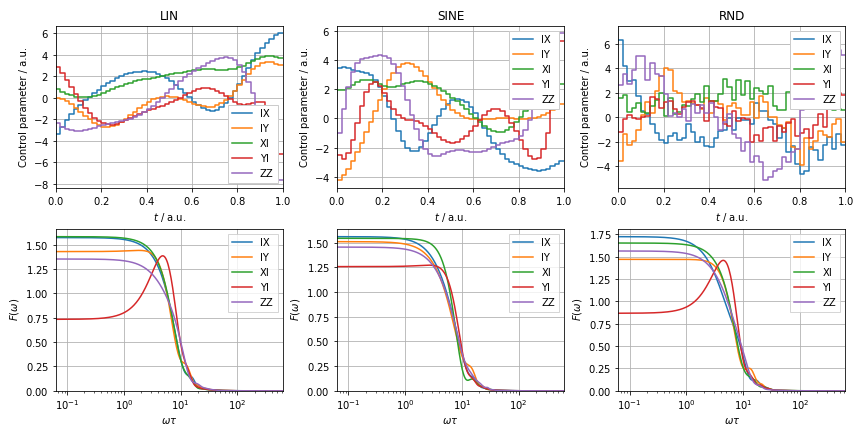# 1.6. Calculating filter functions of optimized gates

In this example we want to optimize a Quantum Fourier Transform gate using QuTiP and compute the filter functions for the optimized gate for different initial pulse amplitudes.

For more information on the QuTiP optimization, see the tutorials here and, for QFT in particular, this notebook.

:

import numpy as np
import qutip as qt
from qutip.control import grape, pulseoptim
from qutip.qip.algorithms import qft

import filter_functions as ff

%matplotlib notebook


We use GRAPE to optimize the pulse for an IQ toy model with $$\sigma_{x,i}$$ and $$\sigma_{y,i}$$ as well as an exchange coupling $$\sigma_z\otimes\sigma_z$$ as controls.

:

# No constant Hamiltonian
H_d = qt.tensor(qt.sigmaz(), qt.sigmaz())*0.0

H_c = [
qt.tensor(qt.sigmax(), qt.qeye(2)),
qt.tensor(qt.sigmay(), qt.qeye(2)),
qt.tensor(qt.qeye(2), qt.sigmax()),
qt.tensor(qt.qeye(2), qt.sigmay()),
qt.tensor(qt.sigmaz(), qt.sigmaz())
]
n_ctrls = len(H_c)
# start point for the gate evolution
U_0 = qt.identity(4)
# Target for the gate evolution - Quantum Fourier Transform gate
U_tar = qft.qft(2)

evo_time = 1
n_ts = 50

# Some optimization parameters
fid_err_targ = 1e-10
max_iter = 10**5
max_wall_time = 10**3

# Initial pulse amplitudes, 'RND'|'LIN'|'ZERO'|'SINE'|'SQUARE'|'TRIANGLE'|'SAW
p_types = ['LIN', 'SINE', 'RND']
results = {
p_type: pulseoptim.optimize_pulse_unitary(
H_d, H_c, U_0, U_tar, n_ts, evo_time, fid_err_targ=fid_err_targ,
max_iter=max_iter, max_wall_time=max_wall_time,
out_file_ext=None, alg='GRAPE', init_pulse_type=p_type, gen_stats=True,
phase_option='PSU',  # ignore global phase
) for p_type in p_types
}


We can now easily set up PulseSequence instances from the final amplitudes and compute the filter functions of each pulse:

:

import matplotlib.pyplot as plt
from filter_functions import plotting

identifiers = ['XI', 'YI', 'IX', 'IY', 'ZZ']
pulses = {
p_type: ff.PulseSequence(
list(zip(H_c, result.final_amps.T, identifiers)),
list(zip(H_c, np.ones((n_ctrls, n_ts)), identifiers)),
[evo_time/n_ts]*n_ts
) for p_type, result in results.items()
}

fig, ax = plt.subplots(2, 3, figsize=(12, 6))
for i, (p_type, pulse) in enumerate(pulses.items()):
# Plot the pulse train
*_, = plotting.plot_pulse_train(pulse, fig=fig, axes=ax[0, i])
ax[0, i].set_title(p_type)
# Plot the filter functions
omega = ff.util.get_sample_frequencies(pulse, spacing='log')
*_, = plotting.plot_filter_function(pulse, omega, fig=fig, axes=ax[1, i])

fig.tight_layout()Evidently, the pulses with linear and random initial amplitudes filter out DC noise better than those with sinusoidal initial amplitudes, in particular for $$\sigma_y$$ noise on the first qubit. There, the filter function has DCG-like character to an extent.